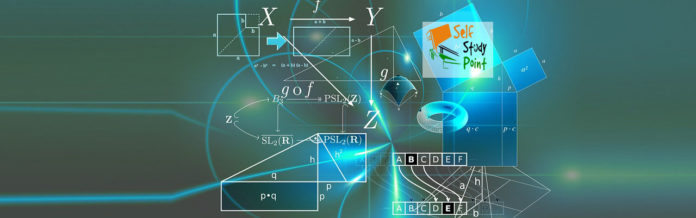### Simple Microscope or Magnifying glass:

The Magnifying power of a simple microscope is defined as the ratio of the angles subtended by the image and the object on the eye when both are at the least distance of distinct vision from the eye.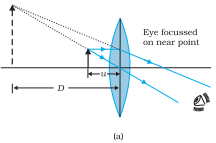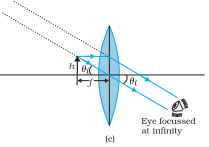Fig. A simple microscope; (a) the magnifying lens is located such that the image is at the near point, (b) the angle subtended by the object, is the same as that at the near point, and (c) the object near the focal point of the lens; the image is far off but closer than infinity

The linear magnification m, for the image formed at the near point D, by a simple microscope can be obtained by using the relation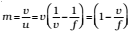Now according to our sign convention, v is negative and is equal in magnitude to D.

Thus, the magnification is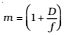### Compound Microscope:

It consists of 2 converging lenses. The magnifying power of the compound microscope is defined as the ratio of the angle subtended at the eye by the final image to the angle subtended at the eye by the object when both the final image and the object are situated at the least distance of distinct vision from the eye.

We now obtain the magnification due to a compound microscope. The ray diagram of Fig. shows that the (linear) magnification due to the objective, namely h′/h, equals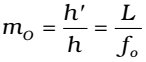Where we have used the resultHere h2 is the size of the first image, the object size being h and fo being the focal length of the objective. The first image is formed near the focal point of the eyepiece. The distance L, i.e., the distance between the second focal point of the objective and the first focal point of the eyepiece (focal length fe) is called the tube length of the compound microscope.

When the final image is formed at the near point, is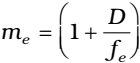When the final image is formed at infinity, the angular magnification due to the eyepiece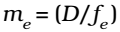Thus, the total magnification [(according to above eq], when the image is formed at infinity,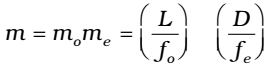Clearly, to achieve a large magnification of a small object (hence the name microscope), the objective and eyepiece should have small focal lengths. In practice, it is difficult to make the focal length much smaller than 1 cm. Also, large lenses are required to make L large.